# Physical Quantities, Units and Measurements Quiz 2

#### Quiz Description

In this second quiz on Physical quantities, units, measurements, and dimensions, We shall go deeper in the study of the respective concepts. You shall be further tested on the identification of measurement parameters, calculation of error, and your understanding of SI units among others. It is going to be beneficial to take this second quiz as it’s going to boost your reasoning.

A physical quantity could be defined as a body or material property that can be quantified and measured. After quantification and measurement, the final results need to be expressed with respect to some standards, and this is when units come in. Units are standards for measurement. Some examples of units are: kilogram (kg), meter(m), Ampere(A), etc. Physical quantities can also be further divided into vectors (physical quantities with magnitude and direction) and scalar quantities (physical quantities with magnitude but no direction).

Answering the following questions correctly means you’re fit for the task. Here is your chance to prove your strength by solving the quiz. We wish you the best as you practice alongside other exciting physics quizzes

When there is no wind, the engines of an airship push it due north at 20 m / s.

The wind is blowing from the west at 12 m / s.

Which vector diagram correctly shows how the resultant velocity R of the airship is obtained?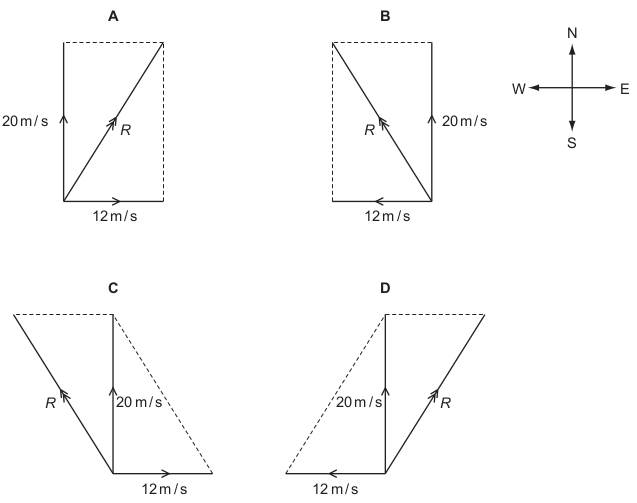Correct
• A
• B
• C
• D

A length of copper pipe, of uniform cross-section and several meters long, carries water to a tap.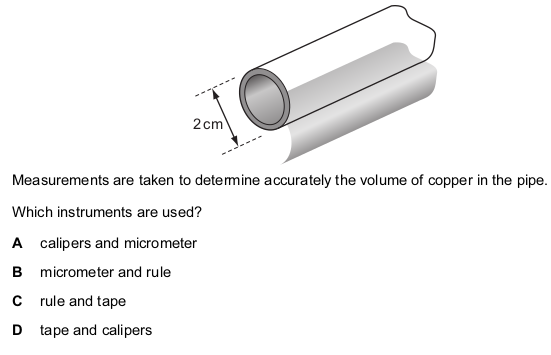Correct
• A
• B
• C
• D

A manufacturer measures accurately the dimensions of a wooden floor tile.

The approximate dimensions of the tile are shown.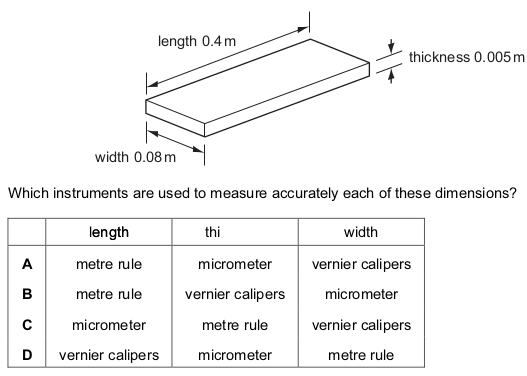Correct
• A
• B
• C
• D

Which pair of quantities includes one scalar and one vector?

Correct
• mass-time
• temperature-time
• temperature-velocity
• velocity-weight

A reel of copper wire is labelled ‘length 30 m’ and ‘diameter 2 mm’. A student calculates the volume of the copper wire.

Which instruments does he use to measure accurately the length and the diameter of the wire?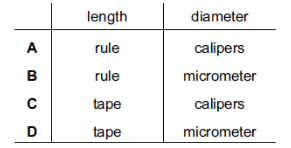Correct
• A
• B
• C
• D

Which row correctly shows examples of a vector quantity and a scalar quantity?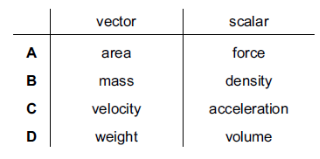Correct
• A
• B
• C
• D

Vernier calipers read to one tenth of a millimeter.

Which reading is given to this precision?

Correct
• 3.3 cm
• 3.31 cm
• 3.310 cm
• 3.312 cm

Velocity is given by the change in displacement divided by the change in time.

How many vector quantities appear in this statement?

Correct
• 0
• 1
• 2
• 3

The level of water in a measuring cylinder is 75 cm 3 . A stone of volume 20 cm 3 is lowered into the water.

Correct
• 20 cm 3
• 55 cm 3
• 75 cm 3
• 95 cm 3

A plumber measures, as accurately as possible, the length and internal diameter of a straight copper pipe.

The length is approximately 80 cm and the internal diameter is approximately 2 cm.

What is the best combination of instruments for the plumber to use?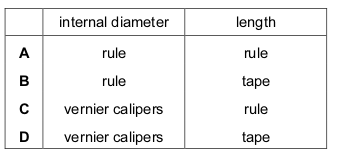Correct
• A
• B
• C
• D

What is the correct unit for the quantity shown?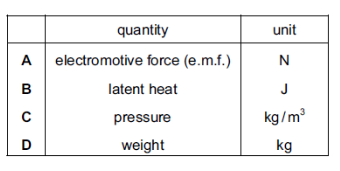Correct
• A
• B
• C
• D

The diameter and the length of a thin wire, approximately 1 m in length, are measured as accurately as possible.

What are the best instruments to use?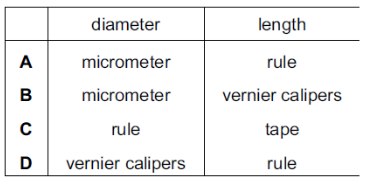Correct
• A
• B
• C
• D

A quantity is quoted as having a value of 6.2 ms. In what units is it measured?

Correct
• meters
• meters per second
• microseconds
• milliseconds

Power is measured in watts.

What is the correct symbol for millions of watts?

Correct
• mw
• mW
• Mw
• MW

Vernier calipers are shown with the jaws closed.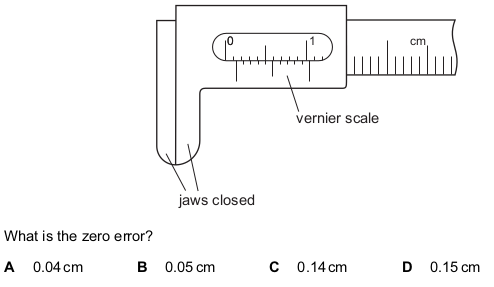Correct
• A
• B
• C
• D

Which instrument is most easily used to measure the internal diameter of a pipe?

Correct
• manometer
• measuring cylinder
• micrometer
• vernier calipers

Which statement about scalars and vectors is correct?

Correct
• A scalar has direction but no size
• A scalar has size but no direction.
• A vector has direction but no size.
• A vector has size but no direction.

Which list contains only scalar quantities?

Correct
• acceleration, displacement, mass
• acceleration, distance, speed
• displacement, mass, velocity
• distance, mass, speed

The following statements are about motion.

1 A plane flies due East for 600 km.

2 A runner’s average speed in a race around a track is 5 m / s.

3 A snail crawls at 3 mm / s in a straight line towards a lettuce.

4 A tourist travels 500 km on a journey.

Which statements describe vector quantities?

Correct
• 1 and 2
• 1 and 3
• 2 and 3
• 2 and 4

Which is the correct statement about force and velocity?

Correct
• Force and velocity are both scalars.
• Force and velocity are both vectors.
• Force is a scalar, velocity is a vector.
• Force is a vector, velocity is a scalar.

Which of the following groups of physical quantities consists only of scalars?

Correct
• acceleration, force, velocity
• acceleration, mass, speed
• force, time, velocity
• mass, speed, time